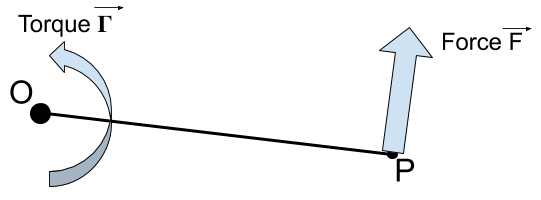👋 Hello! I'm Alphonsio the robot. Ask me a question, I'll try to answer.

# How to convert Newton meter to NewtonThe formula to calculate the force created by a given torque is:

﻿﻿

With :

• ﻿﻿ is the force expressed in newtons [N]
• ﻿﻿ is the torque expressed in newtons-meters [N.m]
• ﻿﻿ is the radius expressed in meters [m], note that ﻿﻿ , the length of the OP segment.

This formula can also be expressed in the general case with the cross product:

﻿﻿

With :

• ﻿﻿ is the force vector expressed in newtons [N]
• ﻿﻿ is the torque vector expressed in newtons-meters [N.m]
• ﻿﻿ is the vector from rotation center ﻿﻿ to the point ﻿﻿ where the force ﻿﻿ is applied

More